﻿ DP Formulation for Distribution System Planning Considering Distributed Energy Resources and Market EnvironmentsPublications are Open
Access in this journal
Article Versions
Export Article
• Normal Style
• MLA Style
• APA Style
• Chicago Style
Research Article
Open Access Peer-reviewed

### DP Formulation for Distribution System Planning Considering Distributed Energy Resources and Market Environments

Nguyen Minh Y
International Transaction of Electrical and Computer Engineers System. 2017, 4(2), 62-67. DOI: 10.12691/iteces-4-2-3
Published online: December 08, 2017

### Abstract

This paper presents a new formulation for the optimal planning of distribution systems considering both the implementation of distributed energy resources and the existence of electricity markets. The problem is to minimize the total cost while subject to the system constraints. The costs incurred during the planning time such as capital cost, replacement cost, and fuel cost, etc. are reverted to the initial stage by the rate of return and summed up to form the objective function, called Net Present Cost. The problem is formulated in Dynamic Programming framework and solved by Dynamic Programming backward algorithm. The numerical simulation in case study shows that the proposed method is capable of providing a flexible planning scheme, i.e., optimal policy while handling all system requirements throughout the project lifetime. In addition, the drawback of Dynamic Programming such as computation time is acceptable in the planning problems.

### 1. Introduction

Recently, Distributed Energy Resource (DER) has emerged to be an important power source to meet the increasing demand of electric power systems. Encompassing a variety of primary resources such as wind, solar and/or fossil fuels, etc., DER is capable of providing electric power at a lower cost and higher level of quality and reliably 1. However, the proliferation of DER particularly in distribution systems, on the other hand, also poses many difficulties to system operators. It is because the distribution system has been designed to be a passive network, i.e., there is no or negligible active power sources inside. The network is fed by the transmission system to supply the electric users. Therefore, all operation, protection and control scheme, etc. are designed to work with uni-directional power flows 2, 3. In addition, nowadays, the electric power industry has been restructured in which the ownership and operation of power systems are decentralized and done through competitive electricity markets 4. Each entity would act for its own benefit rather than for the well-being of the whole system. This would further challenge the system operator since the view point of system-users changes from the system to individual perspective.

Many study efforts have been paid to address the issue of DER in distribution systems and electricity markets. The concept of a new distribution system to integrate DER such as Microgrid, is presented and studied in 5, 6, 7, 8. In this form, all DER’s advantage is expected to be captured while the negative impact can be avoided. The planning of distribution system with DER and/or under market environments is studied in 9, 10, 11. These works mainly focus on upgrading the electric network taken into account the possibility of DER sources existing in some nodes and/or the change of load profiles.

In this paper, we focus on the resource planning for distribution systems under market environments. With the development and maturing of DER technologies, distribution companies have many choices to fulfill their load, either by purchasing electricity from the market and/or building their own DER sources. The distribution companies need to determine the technology, capacity and time that DER sources will be built and operated during the project lifetime. The objective is to minimize the total cost incurred during the project lifetime: Capital cost, Operation and Maintenance (OM) cost, replacement cost and fuel cost; these are reverted to the initial stage by the rate of return and summed up, called Net Present Cost (NPC). The problem is formulated in Dynamic Programming (DP) framework in which the system statuses, decisions and uncertainties are represented by state variables, control variables and random variables. By thus, the problem become a standard DP problem and can be solved by DP backward algorithm. The numerical simulation in case study will show the effectiveness of the proposed planning scheme.

The remainder of this paper is organized as follows. Section 2 presents the mathematical modeling of the objective function, i.e., NPC. Section 3 gives the problem formulation with the technical constraints. Section 4 is for Dynamic Programming (DP) method and DP backward algorithm. Section 5 is to illustrate the application of the proposed scheme in case study. Finally, remarkable points are summarized in Conclusion.

### 2. Economic Evaluation

To evaluate the potential benefit of resource planning, an economic index named Net Present Cost (NPC) is used which represents the total costs incurred during the project lifetime reverted to the initial stage. The NPC is calculated as follows.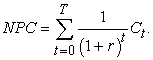(1)

As aforementioned, nowadays, distribution companies have many choices to fulfill their load. Firstly, the electricity can be purchased from the market and supplied end-users via the traditional distribution network. With this choice, the electric network and connections to the transmission system need to be upgraded for handling the increasing amount of loads. The cost to purchase electricity is paid according to the market price. Secondly, distribution companies can plan their own sources (DER) to supply the load thoroughly or partially combined with the purchase from the electricity market. This requires an additional cost for investment, operation and maintenance of the sources; and more importantly, a fuel cost that depends on the technology and actual amount of generations. Therefore, the net cost flow Ct is the sum of the cost of all sources at time t, including capital cost, replacement cost, O&M cost, fuel cost and/or payment for purchasing electricity from the market.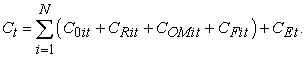(2)

The cost in (2) can be categorized into Fixed Cost (FC) and Variable Cost (VC). FC is the cost that depends only on the capacity of sources, and rarely on generation levels: Capital cost and replacement cost. In planning, the capital cost is calculated based on the average plant cost (cost per unit of capacity setup). Replacement cost, i.e., some parts of the plant degrades faster than others and need to be replaced before the project ends, is estimated as a percentage of the capital cost. VC is the cost that strictly related to the operation of the sources: Fuel cost and energy cost. In planning, the fuel cost is calculated as product of the average fuel cost and output; the energy cost is the purchased electricity multiply with the market price. OM is slightly different and consists of both fixed and variable parts. For convenience, the variable part is added in fuel costs by modifying average fuel costs. Therefore, the net cost flow Ct in (2) can be written in details as: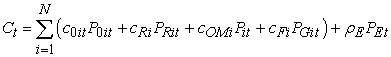(3)

It is worthwhile to note that the O&M cost is the annual payment for operating and maintaining the sources, while the replacement cost only takes place when needed. The amount of generation (PG) and/or purchase (PE) needs to be evaluated according to the demand which is obtained by load forecasting tools. This will be discussed in Section 3.

### 3. Mathematical Formulation

The optimal planning problem is to determine the capacity and time that DER and/or the connection to transmission system need to be built to fulfill the demand. The solution is subject to the technical constraint and the satisfaction of electric users. It is formulated as follows.

Objective function: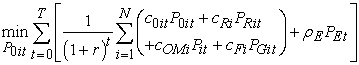(4)

Subject to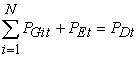(5)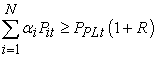(6)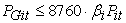(7)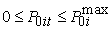(8)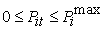(9)

In (4), the objective function is written as a function of the decision variables, P0it, the planning capacity of i-th generation at time t and other cost parameters. Eq. (5) is the constraint of energy balance between the supply and demand in stage t. Eq. (8) is the reserve constrain of distribution systems; typically, the reserve requirement is 10%. Eq. (9) expresses the generation limits according to the capacity factor. Finally, Eq. (6) and (7) shows the planning capacity limits of i-th generation. The replacement capacity can be determined as follows.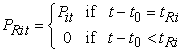(10)

In the above formulation, it can be seen that the capital cost, replacement cost, and OM cost can be evaluated straightforwardly according to the amount of planning, P0it and Pit. However, the cost of fuels and/or electricity purchase needs more detail analysis of the system operation. The economic operation tool such as Unit Commitment (UC) and/or Economic Dispatch (ED) is included to estimate the generated electricity of each source and also, the amount purchased from markets. In planning, it is determined based on the load duration curve, i.e., showing the time duration of each load level. Each source is committed to fulfill a certain area of loads following the rule of least-cost operation, i.e., the source with lower fuel cost will be committed to supply the load first. In this case, the purchase from the transmission system can be thought as a source with the fuel cost is equal the market price. If all the sources are in the increasing order of fuel cost, their commitments to fulfill the demand are expressed in Figure 1 as follows.

• Figure 1. The load duration curve and the commitment of the sources

It is noted that Renewable Energy Source (RES) such as Wind Power (WP), Photovoltaics (PV), etc. consumes no fuel, therefore, they are allowed to generate as much as they can depending on the primary resources.

### 4. Dynamic Programming Method

DP is widely used in power engineering problems such as UC, ED, resource allocation, etc. Particularly, it is an effective method to deal with the uncertainty, nonlinearity and inter-related (i.e., the current decision will affect the optimality of the future decision) problems 5, 13, 14. Therefore, we recommend DP method to solve the optimal planning problem as formulated above.

4.1. Dynamic Programming Framework

Let us define

ut be the planning capacity of sources at the beginning of stage t.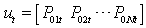(11)

xt be the existing capacity of sources in stage t.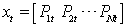(12)

We have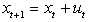(13)

wt be the uncertainty in stage t, such as the uncertainty of load forecasting, electricity price, etc.

We can rewrite the problem in DP framework as follows.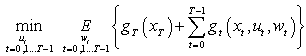(14)

s.t.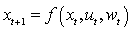(15)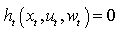(16)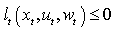(17)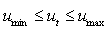(18)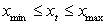(19)

In DP framework, the objective function is formulated in term of three variables: control variable, ut, state variable, xt, and random variable, wt. In (14), the cost function g(xt,ut,wt) is the same as expressed in (4). Eq. (15) shows the stage transition, i.e., the relationship of the current state with the decision in the previous stage as in (13). Eq. (16) - (19) described the equality and inequality constraint such as energy balance, reserve requirement and the capacity limits of sources which are detailed in (5) - (9). The DP framework is shown in Figure 2.

• Figure 2. The problem in DP framework
4.2. Dynamic Programming Backward Algorithm

The DP backward algorithm, as its name means starts from the last stage and moves backward stage-by-stage to the first one. In each stage, a sub-problem that is searching for the optimal decision started from the current state are solved; also, a performance index called cost-to-go function is defined which indicates the minimum added cost of the whole remaining stages. Therefore, it can be recognized that the manipulation in each stage would provide information of how best the performance can be in future. The optimal control at each stage is archived to construct the control policy, μt(xt). The control policy is a function of the system state which gives the optimal decision, i.e., the optimal planning of DG sources at each stage for a given current state xt (the existing capacity of DG technologies). The algorithm will terminate if the following condition is satisfied: (1) the initial stage was reached and (2) the control policy of all possible states was defined [Figure 3]. The DP backward algorithm can be described mathematically as follows.

• Figure 3. Dynamic Programming backward algorithm

### 5. Case Study

In this section, we will apply the proposed method for resource planning of a distribution system with the project lifetime of 10-year. All the potential generation sources have been investigated and taken into considerations: WP, PV, diesels and purchasing from the electricity market. Therefore, the planner needs to decide which kind of sources should be included, how much and when they should be built during the planning time.

Suppose that a load forecasting technique is employed to estimate the electricity demand and load duration in the whole project lifetime. Table 1 shows the electricity demand forecasted in the first year of planning duration; it is expected to increase by 10% each following year.

The potential capacity and cost data of generation sources in the distribution systems are investigated and given in Table 2.

The DP backward algorithm for solving the problem is coded in Matlab software consisting of four modules: Data-input, state-encoding, cost-functions, and the main body is DP backward algorithm. The optimal planning for the distribution system is shown in Figure 4.

• Figure 4. The optimal planning of the distribution system in the case of study

The simulation result shows that all DG sources are planned at different stages of the project lifetime: 1000-kW WP is planned in the first year; PV are planned with 250 kW, 500 kW and 250 kW in the fourth, eighth and ninth year, respectively; and diesels are built with 1750 kW, 500 kW and 1000 kW in the fifth, ninth and tenth year, respectively. It is because WP seems to be the most cost-effective technology with low capital cost, no fuel, and the capacity factor is acceptable. Therefore, the maximum potential of WP is planned in the first year to take its advantage of economy. PV, on the other hand, suffer from high capital cost and low capacity factor but provide the planner with flexibility. Therefore, small amounts of PV are built in different years which mainly to fulfill the increment of loads and reserve requirement (10%) [Figure 5].

• Figure 5. The reserve and capacity factor of the planning during the project lifetime.

Diesels, although incurred an expensive fuel cost, it is cheap in investment. Moreover, the potential capacity is not limited to the resources as that of WP and PV. Therefore, it is planned with a significant amount to serve the demand. About the connection to the transmission line (Trans.), it is suffered from very high capital cost; thus, it is not the choice in this case of study.

The capacity factor and reverse of the system during the planning time are shown in Figure 5. It can be seen that the reserve is maintained above the requirement (10%) throughout the project lifetime. It rises at the first and fifth year since a significant amount of sources is built (WP and diesels). However, at the same time, this also makes the capacity factor decreased. The capacity factor of the distribution system in the planning is from 0.35 to 0.46; this is mainly because of the low load factor, i.e., load profile. The costs incur during the project lifetime is expressed in the following figure [Figure 6].

• Figure 6. The costs incurred during the lifetime of the project

It can be seen that the capital cost is equivalent with the capacity planned during the project while the OM cost is depending on the existing capacity available in the distribution system. The fuel cost rises constantly during the project lifetime because of the increase in the load demand. The rate of increase is reduced at some stages when fuel-free sources are built (WP and PV). In the end of the project, there is a return value. That is the value of the source still in service (individual lifetime) when the project ends. In this case of study, the NPC of the resulting optimal planning is 7.6329 (106 \$).

### 6. Conclusion

This paper has presented a new formulation for the optimal planning of distribution systems. The problem is concerned with the implementation of DER in distribution levels and the availability of electricity markets; the system operator is provided with flexibility to fulfill the load demand. In addition, DER is usually modulated in a small size; this would give the planner even more choices: the capacity and time when the DER is built. Therefore, the planning problem becomes complicated and needs an appropriate solution method. In this paper, we have proposed DP framework and DP backward algorithm to formulate and solve for the resource planning of distribution systems. The numerical simulation in case study has shown that various DER sources need to be considered differently according to their economic and technical properties. Some of them should be built earlier to take the economic advantages (WP), while the other depends on the actual need of the system (i.e., the demand) and/or other technical aspects. One of advantage of DP is to treat the uncertainty during the planning time; however, it was not included in the content of this paper. We expect to address the uncertainty relevant to the planning problem in future work.

### Acknowledgements

This work is supported by Thai Nguyen University of Technology as parts of its project on Smart Grid and Distributed Generation.

### Nomenclature

t: the stage index in the project lifetime, [pu]

t0: the planning, i.e., setup, time of the sources, [pu]

tR: the time (duration) of replacement, [year]

N: the total number of sources, [pu]

i: the number index of DG technologies, [pu]

r: the rate of return, or discount rate, [%]

Ct: the net cost flow, i.e., net cost at stage t, [\$]

C0it: the capital cost of DG technology i at stage t, [\$]

CRit: the replacement cost of DG technology i at stage t, [\$]

COMit: the operation and maintenance cost of DG technology i at stage t, [\$]

CFit: the fuel cost of DG technology i at stage t, [\$]

CEt: the energy cost, i.e., the purchase from electricity market at stage t, [\$]

c0i: the average plant cost of DG technology i, [\$/kW]

cRi: the average replacement cost of DG technology i, [\$/kW]

cOMi: the average operation and maintenance cost of DG technology i, [\$/kW]

cFi: the average fuel cost of DG technology i, [\$/kWh]

ρE: the electricity price, [\$/kWh]

Pit: the existing capacity of DG technology i at stage t, [kW]

PRit: the replacing capacity of DG technology i at stage t, [kW]

P0it: the planning capacity of DG technology i at stage t, [kW]

PGit: the generating electricity of DG technology i at stage t, [kWh]

PEt: the purchasing electricity at stage t, [kWh]

α: the utilization factor, [pu]

β: the capacity factor, [pu]

PLt: the electric load at stage t, [kW]

PPLt: the peak load at stage t, [kW]

R: the rate of reserve requirement, [%]

PDt: the electricity demand at stage t, [kWh]

xt: the state variable, i.e., the existing capacity in stage t, [kW]

wt: the random variable, i.e., the uncertainty occurred in stage t, [pu]

f(): the state transition of the system

ht(): the equality constraint at stage t

lt(): the inequality constraint at stage t

ut: the control variable, i.e., the planning capacity at the beginning of stage t, [kW]

umin, umax : are the control limits of the system

xmin, xmax : are the state limits of the system

J: the cost-to-go function, [\$]

### References

  Ackerman T. G. Anderson G. and L. Soder, “Distributed generation: A definition,” Electric Power System Research, Vol. 57, pp. 195-204, 2001. In article View Article  IEEE Standard 1547, Standard for Interconnecting Distributed Resources with Electric Power Systems, Standards Coordinating Committee, 2003. In article  E. J. Coster, J. M. A. Myrzik, B. Kruimer, and W. L. Kling, “Integration issues of distributed generation in distribution grids,” in Proceeding of IEEE, vol. 99, no. 1, pp. 28-39. In article View Article  Eric Allen and Marija Illic, Price-based Commitment Decisions in the Electricity Market, Springer, 2006. In article View Article  Luis M. Costa, George Kariniotakis, “A stochastic dynamic programming model for optimal use of local resources in market environments,” Proc. IEEE Conferences, Lausanne, Switzerland, 2007. In article View Article  Lasseter RH, “Microgrid and distributed generation,” Journal of Energy Engineering Vol. 133, pp. 144-150, 2007. In article View Article  Robert P. Piagi, H. Lasseter, “Autonomous control of microgrid,” Proc. IEEE Power Engineering Society General Meeting, 2006, In article View Article  H. Nikkhaijoei, R. H. Lasseter, “Distributed generation interface to the CERTS microgrid,” IEEE Transaction on Power Delivery, Vol. 24, No. 3, pp. 1598-1607, 2009. In article View Article  Sirichai Wattanasophon and Bundhit Eua-arporn, “Power distribution system planning with demand uncertainty consideration,” Journals of Electrical Engineering and Technology, Vol. 3, No. 1, pp. 20-28, 2008. In article View Article  G. H. Moon, S. B. Kong, S. K. Joo, H. S. Ryu and T. H. Kim, “Stochastic Integrated generation and transmission planning incorporating electric vehicle deployment,” Journals of Electrical Engineering and Technology, Vol. 8, No. 1, pp. 1-10, 2013. In article View Article  B. Zeng, J. Zhang, Y. Zhang, X. Yang, J. Dong and W. Liu, “Active distribution system planning for low-carbon objective using Cuckoo search algorithm,” Journals of Electrical Engineering and Technology, Vol. 9, No. 2, pp. 433-440, 2014. In article View Article  T. Loenthiran, Dipti Srinivasan, “Multi-agent coordination for DER in Microgrid, Proceeding in IEEE, 2008. In article View Article  Felix A. Farret, M. Godoy Simoes, Integration of Alternative Source of Energy, IEEE Press, John Willey and Son, Inc. Pubisher. In article View Article  Dimitri P. Bertsekas, Dynamic Programming and Optimal Contril, Vol. I, Athena Scientific, Belmont, Massachusetts. In article View ArticleThis work is licensed under a Creative Commons Attribution 4.0 International License. To view a copy of this license, visit http://creativecommons.org/licenses/by/4.0/